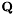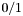# fmpz_poly_q_struct Struct Reference [Type definitions]

Data type for a rational function over the rationals or, equivalently, the integers. More...

#include <fmpz_poly_q.h>

fmpz_poly_p num
fmpz_poly_p den

## Detailed Description

Data type for a rational function over the rationals or, equivalently, the integers.

We represent a rational function overas the quotient of two coprime integer polynomials of type fmpz_poly_t, enforcing that the leading coefficient of the denominator is positive. The zero function is represented as.

## Field Documentation

 fmpz_poly_p fmpz_poly_q_struct::den

Denominator

 fmpz_poly_p fmpz_poly_q_struct::num

Numerator

The documentation for this struct was generated from the following file:
Generated on Wed Dec 8 17:00:29 2010 for FMPZ_POLY_Q by1.6.3